### Overview

Gradient boosting is a technique for regression and classification problems, which produces a prediction model in the form of an ensemble of weak prediction models, typically prediction trees. It builds the model in a stage-wise fashion like other boosting methods do, and generalizes them by allowing optimization of an arbitrary differentiable loss function.

### Motivation

Consider a least-squares regression setting, where the goal is to "teach" a model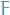to predict values in the form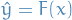by minimizing the mean squared error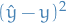, averaged over some training set of actualy values of the output variable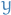.

At each stage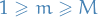, of gradient boosting, it may be assumed that there is a some imperfect model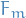. The gradient boosing algorithm improves onby constructing a new model that adds an estimator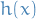to provide a better model: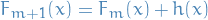.

To find, the gradient boosting solution starts with the observation that a perfectwould imply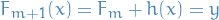i.e. that adding the extra predictorwill make up for all the mistakes our previous model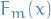did, or equivalently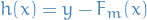Therefore, gradient boosting will fitto the residual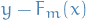. Like in other boosting variants, each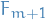learns to correct its predecessor.

The generalization to other loss-functions than the least-squares, comes from the observation thatfor a given model are the negative gradients (wrt.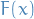) of the squared error loss function, hence for any loss function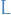we'll simply fit to the negative gradient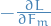.

### Algorithm

Input: training set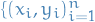, a differentiable loss function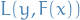, number of iterations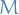.

1. Initialize model with a constant value: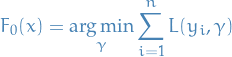2. Forto:

1. Compute so-called psuedo-residuals :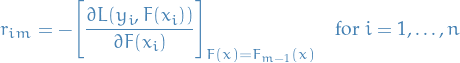1. Fit a base learner (e.g. tree)to psuedo-residuals, i.e. train it using the training set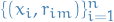2. Compute multiplier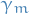sby solving the following one-dimension optimization problem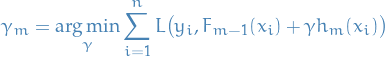1. Update the model: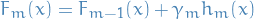3. Output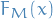• Uses decision trees of fixed size as base learners

### Modified gradient boosting method ("TreeBoost")

• Generic gradient boosting at the m-th step would fit a decision tree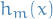to psuedo-residuals
• Let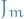be the number of its leaves
• Tree partitions input space intodisjoint regions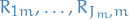and predicts a constant value in each region, i.e.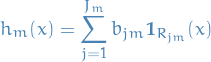where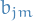is the value predicted in the region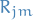.

In the case of usual CART trees, where the trees are fitted using least-squares loss, and so the coefficientfor the regionis equal to just the value of the output variable, averaged over all training instances in.

• Continuing with generic gradient boosting, the model is: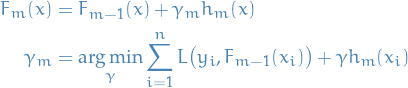The modified gradient tree boosting ("TreeBoost") algorithm is then to instead choose a separate optimal value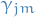for each of the tree's regions, instead of a singlefor whole tree: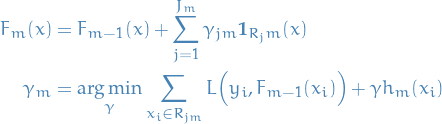where we've simply absorbedinto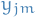.

### Terminology

• Out-of-bag error / estimate is a method for measuring prediction error of methods utilizing bootstrap aggregating (bagging) to sub-sample data used for training. OOB is the mean prediction error on each training sample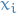, using only the trees that did not havein their bootstrap sample

### Regularization

• Number of trees: Large number of trees can overfit
• Shrinkage: use a modified update rule: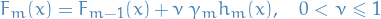where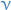is the learnin rate.

• Subsampling is when at each iteration of the algorithm, a base learner should be fit on a subsample of the training set drawn at random without replacement. Sumsample size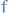is the fraction of training set to use; if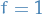then algorithm is deterministic and identitical to TreeBoost
• Limit number of observations in trees' terminal nodes: in the tree building process by ignoring any split that lead to nodes containing fewer than this number of training set instances. Helps reduce variance in predictions at leaves.
• Penalize complexity of tree: model complexity can be defined as proportional number of leaves in the learned trees. Joint optimization of loss and model complexity corresponds to a post-pruning algorithm.
• Regularization (e.g.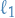,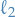) on leaf nodes